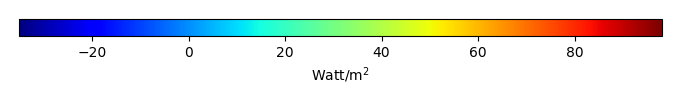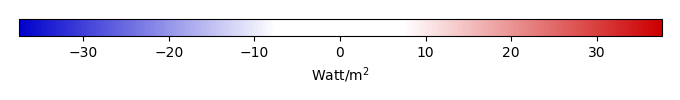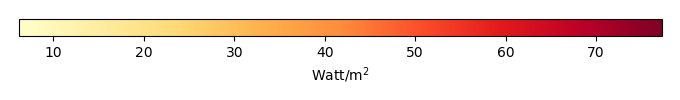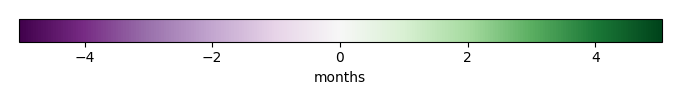# Mean State

Period Mean (original grids) [Watt/m2]
Model Period Mean (intersection) [Watt/m2]
Model Period Mean (complement) [Watt/m2]
Benchmark Period Mean (intersection) [Watt/m2]
Benchmark Period Mean (complement) [Watt/m2]
Bias [Watt/m2]
RMSE [Watt/m2]
Phase Shift [months]
Bias Score 
RMSE Score 
Seasonal Cycle Score 
Spatial Distribution Score 
Overall Score 
Benchmark [-] 44.1
CRUNCEPv7 [-] 52.3 51.6 63.7 44.2 12.4 7.78 20.8 1.29 0.382 0.223 0.806 0.717 0.470
GSWP3v1 [-] 43.5 43.0 49.6 44.2 12.4 -0.924 16.0 1.50 0.390 0.268 0.756 0.887 0.514
WFDEI [-] 54.0 53.4 60.8 44.2 12.4 9.33 16.0 1.09 0.419 0.273 0.837 0.890 0.539
Period Mean (original grids) [Watt/m2]
Model Period Mean (intersection) [Watt/m2]
Model Period Mean (complement) [Watt/m2]
Benchmark Period Mean (intersection) [Watt/m2]
Benchmark Period Mean (complement) [Watt/m2]
Bias [Watt/m2]
RMSE [Watt/m2]
Phase Shift [months]
Bias Score 
RMSE Score 
Seasonal Cycle Score 
Spatial Distribution Score 
Overall Score 
Benchmark [-] 57.5
CRUNCEPv7 [-] 60.0 60.1 87.5 57.5 20.8 2.66 21.1 1.02 0.517 0.380 0.886 0.962 0.625
GSWP3v1 [-] 49.9 50.0 65.5 57.5 20.8 -7.52 20.5 0.609 0.431 0.431 0.955 0.923 0.634
WFDEI [-] 62.9 63.2 78.8 57.5 20.8 5.74 16.9 0.928 0.651 0.430 0.913 0.982 0.681
Period Mean (original grids) [Watt/m2]
Model Period Mean (intersection) [Watt/m2]
Model Period Mean (complement) [Watt/m2]
Benchmark Period Mean (intersection) [Watt/m2]
Benchmark Period Mean (complement) [Watt/m2]
Bias [Watt/m2]
RMSE [Watt/m2]
Phase Shift [months]
Bias Score 
RMSE Score 
Seasonal Cycle Score 
Spatial Distribution Score 
Overall Score 
Benchmark [-] 52.8
CRUNCEPv7 [-] 60.9 60.7 69.0 53.0 19.6 8.02 21.3 0.435 0.501 0.444 0.959 0.893 0.648
GSWP3v1 [-] 55.1 54.9 61.8 53.0 19.6 2.23 18.9 0.441 0.547 0.475 0.959 0.929 0.677
WFDEI [-] 63.8 63.9 64.7 53.0 19.6 11.0 19.3 0.496 0.539 0.470 0.951 0.929 0.672
Period Mean (original grids) [Watt/m2]
Model Period Mean (intersection) [Watt/m2]
Model Period Mean (complement) [Watt/m2]
Benchmark Period Mean (intersection) [Watt/m2]
Benchmark Period Mean (complement) [Watt/m2]
Bias [Watt/m2]
RMSE [Watt/m2]
Phase Shift [months]
Bias Score 
RMSE Score 
Seasonal Cycle Score 
Spatial Distribution Score 
Overall Score 
Benchmark [-] 18.0
CRUNCEPv7 [-] -1.20 -0.937 -5.58 18.1 5.64 -19.3 26.5 0.628 0.455 0.501 0.955 0.474 0.577
GSWP3v1 [-] 14.5 14.9 6.76 18.1 5.64 -3.68 20.8 0.596 0.785 0.475 0.955 0.938 0.726
WFDEI [-] -5.83 -5.52 -11.1 18.1 5.64 -23.9 29.6 0.793 0.388 0.509 0.939 0.282 0.525
Period Mean (original grids) [Watt/m2]
Model Period Mean (intersection) [Watt/m2]
Model Period Mean (complement) [Watt/m2]
Benchmark Period Mean (intersection) [Watt/m2]
Benchmark Period Mean (complement) [Watt/m2]
Bias [Watt/m2]
RMSE [Watt/m2]
Phase Shift [months]
Bias Score 
RMSE Score 
Seasonal Cycle Score 
Spatial Distribution Score 
Overall Score 
Benchmark [-] 22.1
CRUNCEPv7 [-] 28.9 27.5 35.7 22.8 10.8 7.44 20.5 1.79 0.169 0.163 0.733 0.483 0.342
GSWP3v1 [-] 26.9 25.6 32.5 22.8 10.8 5.08 15.0 1.34 0.273 0.233 0.802 0.797 0.468
WFDEI [-] 38.3 37.7 41.0 22.8 10.8 16.0 18.6 1.32 0.170 0.212 0.812 0.946 0.470
Period Mean (original grids) [Watt/m2]
Model Period Mean (intersection) [Watt/m2]
Model Period Mean (complement) [Watt/m2]
Benchmark Period Mean (intersection) [Watt/m2]
Benchmark Period Mean (complement) [Watt/m2]
Bias [Watt/m2]
RMSE [Watt/m2]
Phase Shift [months]
Bias Score 
RMSE Score 
Seasonal Cycle Score 
Spatial Distribution Score 
Overall Score 
Benchmark [-] 27.0
CRUNCEPv7 [-] 12.9 12.9 17.0 27.1 8.81 -14.1 22.5 1.28 0.574 0.535 0.859 0.895 0.679
GSWP3v1 [-] 22.9 22.9 27.6 27.1 8.81 -4.02 15.1 0.642 0.775 0.604 0.933 0.882 0.760
WFDEI [-] 6.76 6.77 13.3 27.1 8.81 -20.2 25.1 1.04 0.471 0.589 0.902 0.706 0.651
Period Mean (original grids) [Watt/m2]
Model Period Mean (intersection) [Watt/m2]
Model Period Mean (complement) [Watt/m2]
Benchmark Period Mean (intersection) [Watt/m2]
Benchmark Period Mean (complement) [Watt/m2]
Bias [Watt/m2]
RMSE [Watt/m2]
Phase Shift [months]
Bias Score 
RMSE Score 
Seasonal Cycle Score 
Spatial Distribution Score 
Overall Score 
Benchmark [-] 28.4
CRUNCEPv7 [-] 37.0 36.6 42.2 28.4 24.4 8.08 20.9 0.919 0.664 0.479 0.897 0.924 0.689
GSWP3v1 [-] 36.4 36.2 40.6 28.4 24.4 7.78 18.9 0.650 0.662 0.536 0.938 0.940 0.722
WFDEI [-] 35.8 35.1 44.0 28.4 24.4 6.50 21.8 0.813 0.606 0.496 0.921 0.794 0.663
Period Mean (original grids) [Watt/m2]
Model Period Mean (intersection) [Watt/m2]
Model Period Mean (complement) [Watt/m2]
Benchmark Period Mean (intersection) [Watt/m2]
Benchmark Period Mean (complement) [Watt/m2]
Bias [Watt/m2]
RMSE [Watt/m2]
Phase Shift [months]
Bias Score 
RMSE Score 
Seasonal Cycle Score 
Spatial Distribution Score 
Overall Score 
Benchmark [-] 40.3
CRUNCEPv7 [-] 53.8 53.9 56.3 40.6 11.0 12.6 23.4 0.392 0.504 0.444 0.973 0.896 0.652
GSWP3v1 [-] 37.8 37.4 41.2 40.6 11.0 -3.37 19.3 0.488 0.515 0.505 0.961 0.936 0.684
WFDEI [-] 48.5 48.4 50.9 40.6 11.0 7.43 18.9 0.439 0.637 0.468 0.969 0.947 0.698
Period Mean (original grids) [Watt/m2]
Model Period Mean (intersection) [Watt/m2]
Model Period Mean (complement) [Watt/m2]
Benchmark Period Mean (intersection) [Watt/m2]
Benchmark Period Mean (complement) [Watt/m2]
Bias [Watt/m2]
RMSE [Watt/m2]
Phase Shift [months]
Bias Score 
RMSE Score 
Seasonal Cycle Score 
Spatial Distribution Score 
Overall Score 
Benchmark [-] 15.2
CRUNCEPv7 [-] -9.23 -8.80 -15.9 15.2 3.78 -24.2 30.4 1.07 0.379 0.489 0.914 0.217 0.498
GSWP3v1 [-] 3.51 3.92 -2.55 15.2 3.78 -11.4 24.3 1.14 0.630 0.452 0.899 0.800 0.647
WFDEI [-] -15.5 -15.1 -21.6 15.2 3.78 -30.5 35.7 1.19 0.302 0.488 0.904 0.158 0.468
Period Mean (original grids) [Watt/m2]
Model Period Mean (intersection) [Watt/m2]
Model Period Mean (complement) [Watt/m2]
Benchmark Period Mean (intersection) [Watt/m2]
Benchmark Period Mean (complement) [Watt/m2]
Bias [Watt/m2]
RMSE [Watt/m2]
Phase Shift [months]
Bias Score 
RMSE Score 
Seasonal Cycle Score 
Spatial Distribution Score 
Overall Score 
Benchmark [-] 56.5
CRUNCEPv7 [-] 66.7 67.3 54.7 56.9 15.0 9.87 17.9 0.507 0.683 0.586 0.962 0.974 0.758
GSWP3v1 [-] 61.4 61.8 53.7 56.9 15.0 4.50 14.8 0.427 0.724 0.646 0.970 0.884 0.774
WFDEI [-] 68.9 69.8 51.9 56.9 15.0 12.1 18.9 0.560 0.626 0.585 0.960 0.983 0.748
Period Mean (original grids) [Watt/m2]
Model Period Mean (intersection) [Watt/m2]
Model Period Mean (complement) [Watt/m2]
Benchmark Period Mean (intersection) [Watt/m2]
Benchmark Period Mean (complement) [Watt/m2]
Bias [Watt/m2]
RMSE [Watt/m2]
Phase Shift [months]
Bias Score 
RMSE Score 
Seasonal Cycle Score 
Spatial Distribution Score 
Overall Score 
Benchmark [-] 20.2
CRUNCEPv7 [-] 23.8 23.6 29.1 20.4 7.08 3.85 21.7 0.553 0.670 0.490 0.933 0.622 0.641
GSWP3v1 [-] 20.3 19.9 30.3 20.4 7.08 0.611 15.7 0.772 0.711 0.616 0.921 0.799 0.733
WFDEI [-] 14.8 14.5 22.9 20.4 7.08 -4.98 20.0 0.552 0.618 0.561 0.946 0.553 0.648
Period Mean (original grids) [Watt/m2]
Model Period Mean (intersection) [Watt/m2]
Model Period Mean (complement) [Watt/m2]
Benchmark Period Mean (intersection) [Watt/m2]
Benchmark Period Mean (complement) [Watt/m2]
Bias [Watt/m2]
RMSE [Watt/m2]
Phase Shift [months]
Bias Score 
RMSE Score 
Seasonal Cycle Score 
Spatial Distribution Score 
Overall Score 
Benchmark [-] 34.3
CRUNCEPv7 [-] 33.4 38.7 11.4 34.6 11.5 4.09 22.9 0.917 0.546 0.457 0.909 0.777 0.629
GSWP3v1 [-] 31.1 36.9 6.93 34.6 11.5 2.33 19.5 0.795 0.586 0.495 0.925 0.916 0.683
WFDEI [-] 32.2 38.0 8.37 34.6 11.5 3.20 22.7 0.854 0.554 0.477 0.921 0.701 0.626
Period Mean (original grids) [Watt/m2]
Model Period Mean (intersection) [Watt/m2]
Model Period Mean (complement) [Watt/m2]
Benchmark Period Mean (intersection) [Watt/m2]
Benchmark Period Mean (complement) [Watt/m2]
Bias [Watt/m2]
RMSE [Watt/m2]
Phase Shift [months]
Bias Score 
RMSE Score 
Seasonal Cycle Score 
Spatial Distribution Score 
Overall Score 
Benchmark [-] 22.7
CRUNCEPv7 [-] 57.0 59.6 54.7 22.8 18.3 37.5 44.0 1.17 0.497 0.505 0.951 0.803 0.652
GSWP3v1 [-] 46.1 49.3 43.1 22.8 18.3 27.3 36.5 1.21 0.513 0.514 0.939 0.787 0.653
WFDEI [-] 55.1 57.5 52.9 22.8 18.3 35.3 41.7 1.19 0.525 0.513 0.936 0.742 0.646
Period Mean (original grids) [Watt/m2]
Model Period Mean (intersection) [Watt/m2]
Model Period Mean (complement) [Watt/m2]
Benchmark Period Mean (intersection) [Watt/m2]
Benchmark Period Mean (complement) [Watt/m2]
Bias [Watt/m2]
RMSE [Watt/m2]
Phase Shift [months]
Bias Score 
RMSE Score 
Seasonal Cycle Score 
Spatial Distribution Score 
Overall Score 
Benchmark [-] 17.0
CRUNCEPv7 [-] -1.15 -0.566 -10.7 17.0 4.51 -18.0 26.8 1.16 0.507 0.492 0.879 0.497 0.573
GSWP3v1 [-] 9.28 9.85 0.800 17.0 4.51 -7.62 22.5 1.05 0.707 0.473 0.896 0.883 0.686
WFDEI [-] -8.84 -8.36 -16.0 17.0 4.51 -25.7 31.8 1.14 0.385 0.505 0.884 0.239 0.504
Period Mean (original grids) [Watt/m2]
Model Period Mean (intersection) [Watt/m2]
Model Period Mean (complement) [Watt/m2]
Benchmark Period Mean (intersection) [Watt/m2]
Benchmark Period Mean (complement) [Watt/m2]
Bias [Watt/m2]
RMSE [Watt/m2]
Phase Shift [months]
Bias Score 
RMSE Score 
Seasonal Cycle Score 
Spatial Distribution Score 
Overall Score 
Benchmark [-] 47.7
CRUNCEPv7 [-] 55.6 54.8 63.2 48.2 15.9 7.02 24.7 0.471 0.506 0.504 0.965 0.765 0.649
GSWP3v1 [-] 50.4 50.0 55.4 48.2 15.9 1.58 22.4 0.487 0.514 0.518 0.955 0.947 0.690
WFDEI [-] 63.4 63.4 64.0 48.2 15.9 13.8 22.3 0.435 0.540 0.511 0.960 0.947 0.694
Period Mean (original grids) [Watt/m2]
Model Period Mean (intersection) [Watt/m2]
Model Period Mean (complement) [Watt/m2]
Benchmark Period Mean (intersection) [Watt/m2]
Benchmark Period Mean (complement) [Watt/m2]
Bias [Watt/m2]
RMSE [Watt/m2]
Phase Shift [months]
Bias Score 
RMSE Score 
Seasonal Cycle Score 
Spatial Distribution Score 
Overall Score 
Benchmark [-] 28.6
CRUNCEPv7 [-] 33.3 33.2 36.7 28.7 12.1 4.82 18.1 1.71 0.618 0.422 0.724 0.800 0.597
GSWP3v1 [-] 31.6 31.4 34.3 28.7 12.1 2.90 15.0 0.815 0.701 0.459 0.899 0.977 0.699
WFDEI [-] 30.8 30.7 33.9 28.7 12.1 2.37 18.8 1.46 0.612 0.402 0.792 0.743 0.590
Period Mean (original grids) [Watt/m2]
Model Period Mean (intersection) [Watt/m2]
Model Period Mean (complement) [Watt/m2]
Benchmark Period Mean (intersection) [Watt/m2]
Benchmark Period Mean (complement) [Watt/m2]
Bias [Watt/m2]
RMSE [Watt/m2]
Phase Shift [months]
Bias Score 
RMSE Score 
Seasonal Cycle Score 
Spatial Distribution Score 
Overall Score 
Benchmark [-] 26.7
CRUNCEPv7 [-] 19.7 19.6 19.7 26.9 8.98 -7.23 20.0 0.736 0.696 0.544 0.925 0.950 0.732
GSWP3v1 [-] 26.2 26.1 25.0 26.9 8.98 -0.725 16.8 0.591 0.800 0.568 0.951 0.940 0.765
WFDEI [-] 15.1 15.0 13.5 26.9 8.98 -11.9 21.6 0.834 0.608 0.548 0.923 0.936 0.713
Period Mean (original grids) [Watt/m2]
Model Period Mean (intersection) [Watt/m2]
Model Period Mean (complement) [Watt/m2]
Benchmark Period Mean (intersection) [Watt/m2]
Benchmark Period Mean (complement) [Watt/m2]
Bias [Watt/m2]
RMSE [Watt/m2]
Phase Shift [months]
Bias Score 
RMSE Score 
Seasonal Cycle Score 
Spatial Distribution Score 
Overall Score 
Benchmark [-] 49.7
CRUNCEPv7 [-] 40.5 40.6 62.9 49.8 11.0 -9.00 17.7 0.415 0.674 0.676 0.969 0.930 0.785
GSWP3v1 [-] 48.2 47.9 69.8 49.8 11.0 -1.90 16.4 0.768 0.740 0.662 0.926 0.894 0.777
WFDEI [-] 50.0 49.8 77.6 49.8 11.0 0.229 17.3 0.649 0.780 0.632 0.944 0.987 0.795
Period Mean (original grids) [Watt/m2]
Model Period Mean (intersection) [Watt/m2]
Model Period Mean (complement) [Watt/m2]
Benchmark Period Mean (intersection) [Watt/m2]
Benchmark Period Mean (complement) [Watt/m2]
Bias [Watt/m2]
RMSE [Watt/m2]
Phase Shift [months]
Bias Score 
RMSE Score 
Seasonal Cycle Score 
Spatial Distribution Score 
Overall Score 
Benchmark [-] 30.2
CRUNCEPv7 [-] 34.2 34.1 51.4 30.3 13.0 4.40 15.8 1.50 0.354 0.206 0.796 0.607 0.434
GSWP3v1 [-] 26.0 25.9 42.2 30.3 13.0 -3.69 14.2 1.58 0.359 0.268 0.776 0.591 0.452
WFDEI [-] 40.2 40.2 47.9 30.3 13.0 10.3 14.9 1.22 0.303 0.269 0.830 0.902 0.515
Period Mean (original grids) [Watt/m2]
Model Period Mean (intersection) [Watt/m2]
Model Period Mean (complement) [Watt/m2]
Benchmark Period Mean (intersection) [Watt/m2]
Benchmark Period Mean (complement) [Watt/m2]
Bias [Watt/m2]
RMSE [Watt/m2]
Phase Shift [months]
Bias Score 
RMSE Score 
Seasonal Cycle Score 
Spatial Distribution Score 
Overall Score 
Benchmark [-] 40.3
CRUNCEPv7 [-] 50.4 50.5 46.3 40.4 9.36 10.2 19.0 0.721 0.466 0.400 0.933 0.697 0.579
GSWP3v1 [-] 43.9 44.2 40.9 40.4 9.36 3.72 16.5 0.707 0.546 0.409 0.929 0.746 0.608
WFDEI [-] 45.5 45.8 40.7 40.4 9.36 5.48 15.5 0.647 0.599 0.409 0.938 0.795 0.630
Period Mean (original grids) [Watt/m2]
Model Period Mean (intersection) [Watt/m2]
Model Period Mean (complement) [Watt/m2]
Benchmark Period Mean (intersection) [Watt/m2]
Benchmark Period Mean (complement) [Watt/m2]
Bias [Watt/m2]
RMSE [Watt/m2]
Phase Shift [months]
Bias Score 
RMSE Score 
Seasonal Cycle Score 
Spatial Distribution Score 
Overall Score 
Benchmark [-] 41.5
CRUNCEPv7 [-] 59.2 58.6 64.2 42.0 6.56 15.8 27.0 0.671 0.597 0.574 0.935 0.939 0.724
GSWP3v1 [-] 65.9 65.1 72.3 42.0 6.56 21.7 34.2 0.485 0.490 0.538 0.959 0.848 0.675
WFDEI [-] 67.8 67.3 74.0 42.0 6.56 23.4 35.6 0.429 0.506 0.523 0.960 0.823 0.667

# Temporally integrated period mean

BENCHMARK MEANMODEL MEANBIASBIAS SCORERMSERMSE SCOREBENCHMARK MAX MONTHMODEL MAX MONTHDIFFERENCE IN MAX MONTHSEASONAL CYCLE SCORESPATIAL TAYLOR DIAGRAMMODEL COLORS# Spatially integrated regional mean

MODEL COLORSREGIONAL MEANANNUAL CYCLEMONTHLY ANOMALYANNUAL CYCLE# All Models

BenchmarkCRUNCEPv7GSWP3v1WFDEI# Data Information

creation_date: Tue Jul 28 15:54:11 PDT 2015

source_file: This product is generated from monthly Global Biosphere Amtospher Flux (GBAF) observations

title: derived GBAF in 0.5x0.5

Approach: I read this variable from data file, and then save the data in NetCDF format by each month and each year. Meanwhile, I also converted the unit from MJoule*m-2*d-1 to Watt/m2.

Temporal resolution: monthly

General information: This product was derived from Monthly estimates of global biosphere-atmosphere fluxes from Biogeochemistry group at Max Planck Institute in Jena, Germany.

Spatial resolution: 0.5x0.5 degree